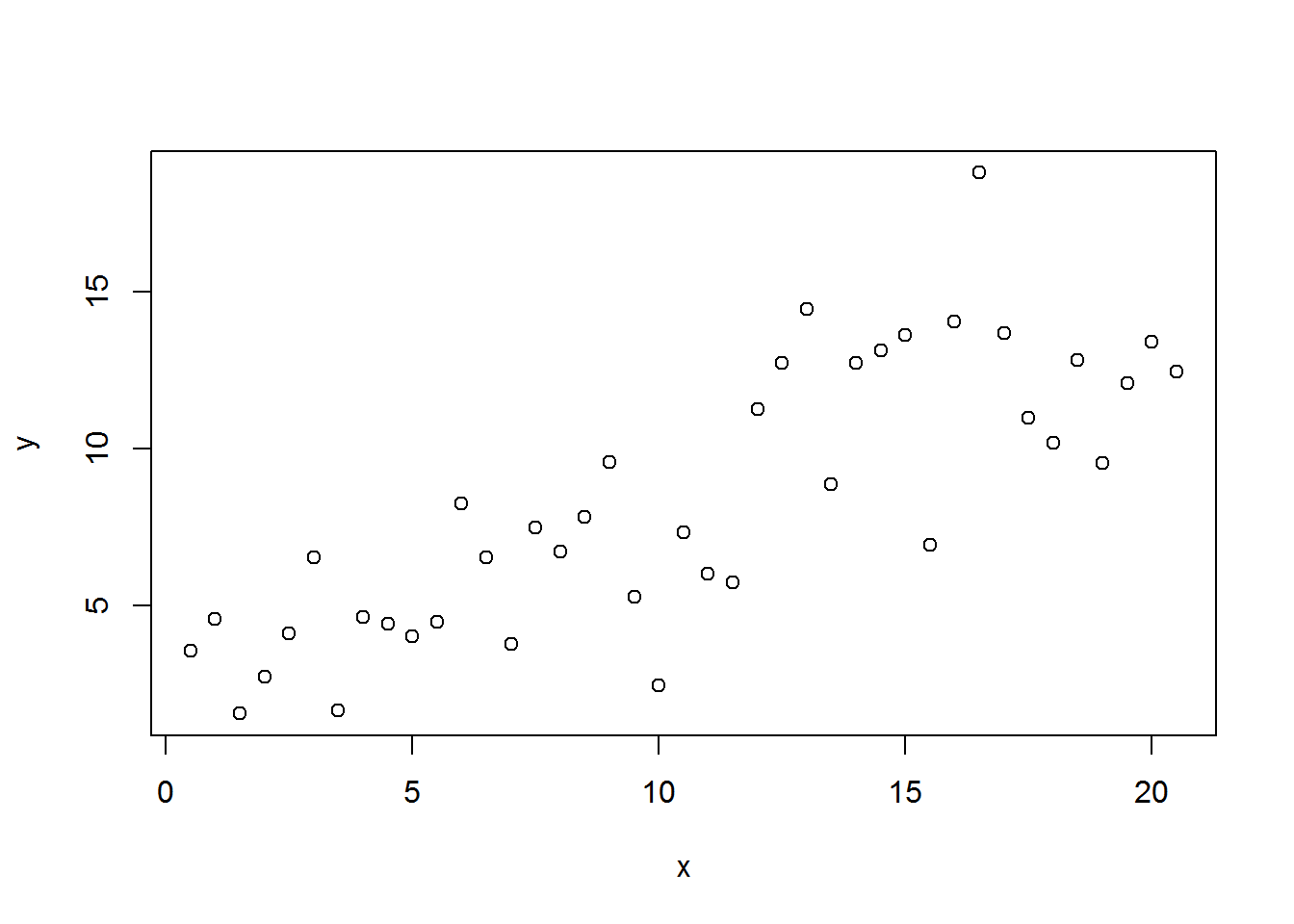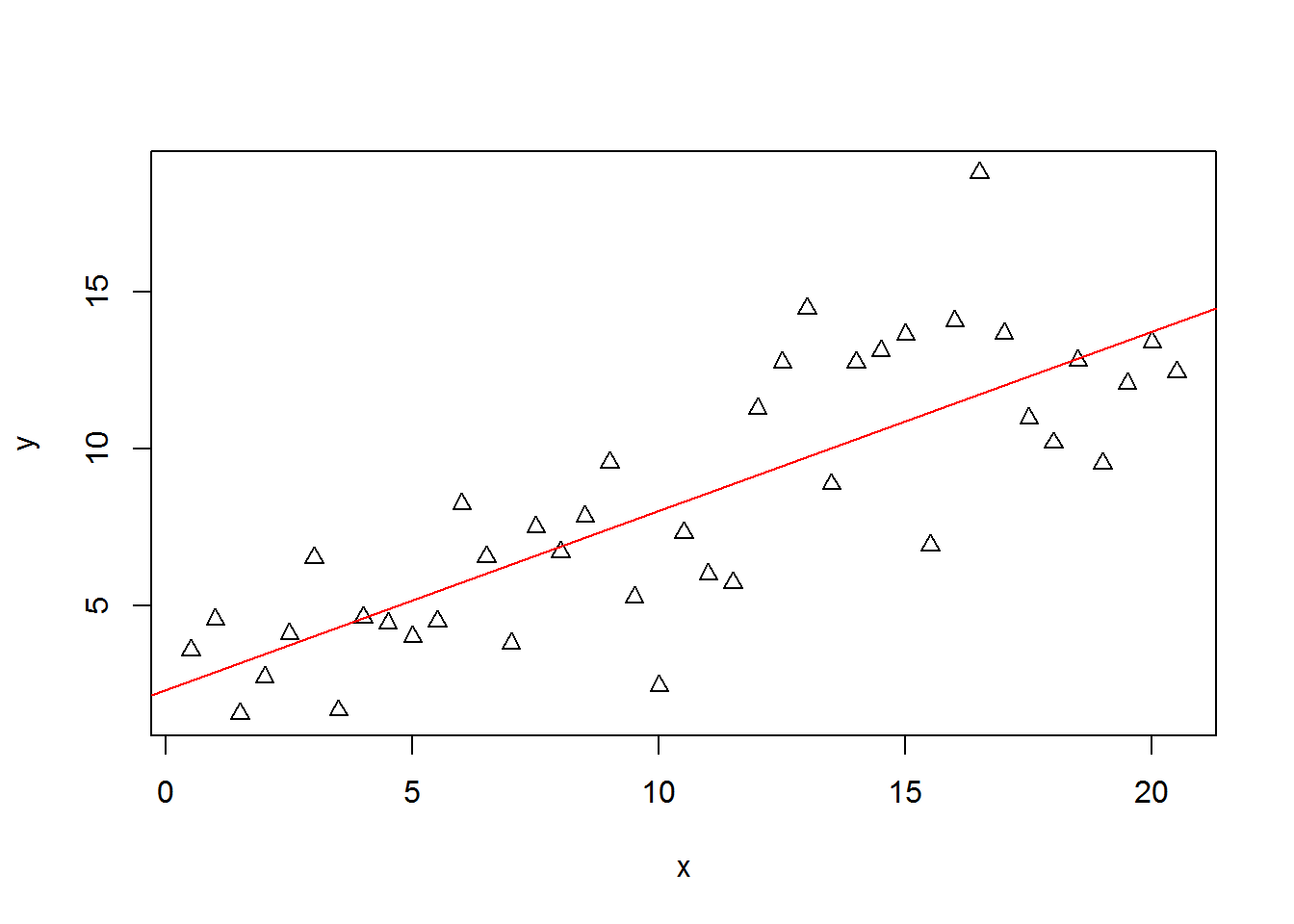A regression analysis is a technique for estimating he values of the coefficients in a model of an economic process. For example, in the labor suply model, we would like to estimate the effect of financial incentives (principally the hourly wage) on the number of hours worked.

# 1- Specification of linear model.

Let assume we want to look at the causal effect of X on Y where X is the only variable that affects Y. In the labor supply model, If we assume only the hourly wage (dependent variable X) matters for the number of hours (independent variable Y) supplied bu the worker. The specification for the linear model is the following

$Y_i = \beta_0 +\beta_1 X_i +\varepsilon_i$

The variables

• Y is called the dependent variable, which is the variable we want to explain the variation.

• X is called the explanatory variable or called indendent variable variable

• is the error term, which can be omitted or unobserved variables.

The coefficients : $$\beta_0$$ and $$\beta_1$$ are parameters of the model, which are fixed values.

• The parameter $$\beta_0$$ is called constant. In the labor supply model, the parameter $$\beta_0$$ is the hours worked with minimum hourly wage.

• The parameter $$\beta_1$$ is the slope or the marginal increase of Y due to X. $\beta_1=\dfrac{\Delta Y}{\Delta X}$

# 2 - Estimation of the Model.

When we believe that some economic behavior is correctly described by simple regression model as above, the values of the parameter $$\beta_0$$ and $$\beta_1$$ are unknown. We will never discovered the true values. However, we can estimate (approximate) these values from available data like the Canadian Labor Force Survey. The data provide the basis for making estimates the unknown parameters.

The Labour Force Survey is a monthly survey which measures the current state of the Canadian labour market and is used, among other things, to calculate the national, provincial, territorial and regional employment and unemployment rates.

$Y_i = \beta_0 +\beta_1 X_i +\varepsilon_i$

## Case 1 : Regress y on x: marginal value

The goal is to estimate the values of $$\hat{\beta}_0$$ and $$\hat{\beta}_1$$ using collected data. To be able to show you how to estimate coefficients, we are using the OLS_example where we have generated variables (x, y, z).

setwd("C:/Users/MamadouYaya/Dropbox/Fall2019/TA_Fall2019/Data")
head(OLSdata)
##     x        y z
## 1 0.5 3.575166 0
## 2 1.0 4.564874 0
## 3 1.5 1.566132 0
## 4 2.0 2.733268 0
## 5 2.5 4.106824 0
## 6 3.0 6.524267 0

The following code import and plot a scatter graph to illustrate the correlation between these continuous variabes.

plot(OLSdata$x,OLSdata$y,ylab = "y",xlab = "x")To estimate the causal effect of X on Y with R, please search on the help meny the following command : lm which means linear model. This function can be used to create a simple regression model.

myols= lm(OLSdata$y ~ OLSdata$x)
summary(myols)
##
## Call:
## lm(formula = OLSdata$y ~ OLSdata$x)
##
## Residuals:
##     Min      1Q  Median      3Q     Max
## -5.5701 -1.5911 -0.1842  2.0964  7.0370
##
## Coefficients:
##             Estimate Std. Error t value Pr(>|t|)
## (Intercept)  2.32212    0.81645   2.844  0.00706 **
## OLSdata$x 0.57086 0.06774 8.427 2.58e-10 *** ## --- ## Signif. codes: 0 '***' 0.001 '**' 0.01 '*' 0.05 '.' 0.1 ' ' 1 ## ## Residual standard error: 2.566 on 39 degrees of freedom ## Multiple R-squared: 0.6455, Adjusted R-squared: 0.6364 ## F-statistic: 71.01 on 1 and 39 DF, p-value: 2.576e-10 The estimate of the parameters from these observations are $$\hat{\beta}_0$$ and $$\hat{\beta}_1$$. In this case, the correspondance line that fit the data is $\hat{y}_i=\hat{\beta}_0 + \hat{\beta}_1 \hat{x}_i$ This is called the estimated regression line. During the conference, I will talk about labor supply to illustrate the estimate of the coefficient. $\hat{y}_i=\hat{\beta}_0 + \hat{\beta}_1 \hat{x}_i$ From the above regression, the estimated coefficients are $$\hat{\beta}_0=2.30755$$ and $$\hat{\beta}_1=0.57086$$. Then the relation between y and x from our data is the following: $\hat{y}_i=2.30755 + 0.57086 \hat{x}_i$ For given value of the independent variable $$x$$, we can predict the correspondent value of the dependent variable (more details during the conference). plot(OLSdata$x,OLSdata$y,ylab = "y",xlab = "x",pch=24) abline(lm(OLSdata$y~ OLSdata$x),col="red")### Interpretation of the slope $$\hat{\beta}_1$$: How to interpret this value ? ### Exercise 1: Let assume we regress number of hours worked per week (noted by$$h_i$$) on his hourly wage (noted by $$\omega_i$$). $h_i = \beta_0 +\beta_1 \omega_i +\varepsilon_i$ If we have the number hours per week of workers living in Montreal and their corresponding hourly rate and you estimate the parameters of the labor supply model. How to interpret $$\beta_0$$ and $$\beta_1$$. ## Case 2 : Regress log(y) on log(x): Elasticity. The elasticity tells us how the proportional change in y, $$\Delta y/y$$, is related to the proportional change in x,$$\Delta y/y$$, at a particular point (as seen in class). $\varepsilon=\dfrac{\Delta y/y}{\Delta x/x}$ Example 1: if the elasticity of labor supply is 1. What this means ? The elasticity can be expressed also as follow: $\varepsilon=\dfrac{\Delta y/y}{\Delta x/x} \equiv \dfrac{\log(y)}{\log(x)}$ To estimate the elasticity of y with respect to x, we have only to regress $$\log(y)$$ on $$\log(x)$$. $\log y_i = \alpha_0 +\alpha_1 \log x_i +\varepsilon_i$ OLSdata$logx=log(OLSdata$x) OLSdata$logy=log(OLSdata$y) myols_elas=lm(OLSdata$logy~OLSdata$logx) summary(myols_elas) ## ## Call: ## lm(formula = OLSdata$logy ~ OLSdata$logx) ## ## Residuals: ## Min 1Q Median 3Q Max ## -1.1723 -0.2314 0.0599 0.2969 0.7572 ## ## Coefficients: ## Estimate Std. Error t value Pr(>|t|) ## (Intercept) 0.87685 0.16799 5.220 6.25e-06 *** ## OLSdata$logx  0.51938    0.07433   6.987 2.23e-08 ***
## ---
## Signif. codes:  0 '***' 0.001 '**' 0.01 '*' 0.05 '.' 0.1 ' ' 1
##
## Residual standard error: 0.4114 on 39 degrees of freedom
## Multiple R-squared:  0.5559, Adjusted R-squared:  0.5445
## F-statistic: 48.82 on 1 and 39 DF,  p-value: 2.23e-08

According to the result in the table, the elasticity of y with respect to x is $$\alpha_1= 0.51938$$. An increase of 1% of x will increase the variable y by 0.51%. Thus, the elasticity of y with respect to y is inelastic.

## Case 2 : x is a dummy variable.

A dummy variable (aka, an indicator variable) is a numeric variable that represents categorical data:

• Gender : Female or Male
• Grade : College or non college
• Age : Old or young
• Immigration : Native or Not native

In the OLSexample database, the variable z is a dummy: the two value are 1 or 0.Each individual (represented by a row) can take be either in group 0 or group 1. To estimate the difference between the two group.

$y_i = \delta_0 + \delta_1 z_i +\varepsilon_i$

• If $$z_i=0$$ then $$y_i (z_i=0) = \delta_0 + \varepsilon_i$$

• If $$z_i=1$$ then $$y_i(z_i=1) = \delta_0 + \delta_1 + \varepsilon_i$$

Assuming the average errors is zero $$\bar{\varepsilon}_i = 0$$, then

• The average value for individuals with $$z_i =0$$ $\bar{y}_i (z_i=0) = \delta_0$
• The average value for individuals with $$z_i =1$$ $\bar{y}_i (z_i=1) = \delta_0 + \delta_1$

• The difference between the two group is $\bar{y}_i (z_i=1)- \bar{y}_i (z_i=1) = \delta_1$

### Illustration : Estimate the parameters using our data where z is a dummy variable.

• Plot a barplot to shows the difference between the two group.
table3 <- tapply(OLSdata$y ,INDEX = OLSdata$z,FUN=mean,na.rm =TRUE)
round(table3,digits = 3)
##      0      1
##  5.012 11.463
• Regress y on z and find the parameters $$\delta_0$$ and $$\delta_1$$
lm(OLSdata$y ~ factor(OLSdata$z))
##
## Call:
## lm(formula = OLSdata$y ~ factor(OLSdata$z))
##
## Coefficients:
##        (Intercept)  factor(OLSdata\$z)1
##              5.012               6.451

### Exercise 2: Example of Gender wage gap

Let assume the variable z is the gender of individual, which take 1 if Male and 0 if Female. The variable y represent the hourly wage of individual.

• What represent the parameter $$\delta_0$$ ?
• What represent the parameter $$\delta_1$$ ?

# 3 - Interpretation of the regression.

### 3 Things matters of the interpretation (More details during the conference)

• The sign of the coefficient.
• The level of significance.
• The measur of the fit

# 4 - Empirical aspects of labor supply.

The supply of labor is probably the area of labor economics in which the greastest number of empirical studies have been carried out during the last three decades. One of the main reasons is that for those whose job, researchers and decision makers, is to implement or plan employment policies or fiscal system, the response of labor supply is a primary consideration.

What would happens if the employers increases the hourly wage ?

What would happens if to unemployment rate if the overtime are taxed ?

What is the consequence of increasing minimum wage on labor supply ?

The estimate of the parameters of the labor supply model is a valuable aid to decision making in matters of public policy. These estimates will allows research to predict the consequences of differents public policies on the individual behaviors (labor supply for workers, family income, women partipation, etc.).

### 4.1 Basic equation of labor supply.

As a general rule, estimates of labor supply equations (labor supply demand) are made on the basis of cross-section data (it is difficult to collect data temporal elements) produced by investigating a large population. For our illustation and assignments, we are using the Canadian Labor Force Survey where we have individuals information.

To estimate the parameters (elasticity) of labor supply model, empirical researchers (econometricians) tries to estimates almost always rest on the basic equation relating hours $$h_t$$ worked by a given individual at hourly wage $$\omega_t$$. The double-log-linear relation is a typical reduced form of this basic equation:

$ln h_t = \alpha_w ln w_t + \varepsilon_t$

Where $$\alpha_w$$ is the parameter to be estimate. This parameter measures the wage elasticity of labor supply. The theoritical model taught us this coefficient is positive.

Estimating the above equation of labor supply will lead probabily to biased estimates, which means $$\hat{\alpha}_w$$ $$\neq$$ $$\alpha_w$$.